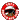Broadcast Sheet
 INDEX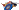Line 1 Basic CarLine 2 Basic CarLine 3 Build CodesLine 4 Build CodesLine 5 Build CodesLine 6 Sales CodesLine 7 Sales CodesLine 8 Sales CodesLine 9 Sales CodesLine 10 Sales CodesLine 11 Sales CodesLine Remarks'71 Charger Super Bee
 CONTACT INFORMATIONJohn M. Bōber  Viera, FL 32955Chrysler Corporation Car Production Broadcast Sheet

Line 4 Build Codes.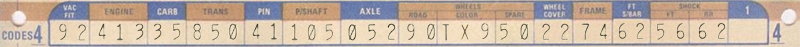Build codes run from line 3, 4 and 5. Most of the numbers are the last few digits of the "factory production" part numbers.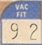VAC FIT: The last two digits of the part number for the power brake vacuum fitting. 01 = 3580201 3-way w/ thermostatic ingnition 02 = 2580202 4-way w/ thermostatic ingnition 04 = 3461304 2-way 11 = 3461304 3-way 23 = 3461323 3-way w/o AC 38 = 1948838 3-way w/ AC 54 = 1944154 1-way 66 = 2405466 2-way .247 tube 92 = 1944092 2-way .122 tube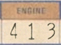ENGINE: The last three digits of the assembly line part number. 382 = 340 4BBL, manual trans., heater, 275hp 383 = 340 4BBL, manual trans., A/C, 275hp 407 = 426 Hemi, manual trans., heater, 425hp 408 = 426 Hemi, auto. trans., heater, 425hp 412 = 440 6BBL, manual trans., heater, 385hp 413 = 440 6BBL, auto. trans., heater, 385hp 418 = 383 4BBL, manual trans., heater, 300hp 419 = 383 4BBL, auto. trans., heater, 300hp 420 = 383 4BBL, auto. trans., A/C, 300hp 443 = 440 4BBL, manual trans., heater, 370hp 444 = 440 4BBL, auto. trans., heater, 370hp 445 = 440 4BBL, auto. trans., A/C, 370hp 451 = 340 4BBL, auto. trans., heater, 275hp 452 = 340 4BBL, auto. trans., A/C, 275hp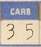CARB: The last two digits of the assembly line part number, not the part number used in catalogs. This number will be used with reference of the engine, transmission, with or with out freash air hood, and with or with out AC. 30 = 383 4bbl Auto w/AC 32 = 440 4bbl 4spd 34 = 440 6pk 4spd w/Ramcharger 35 = 440 6pk Auto w/Ramcharger 42 = 383 4bbl Auto w/Ramcharger 48 = 383 4bbl 4spd 50 = 383 4bbl Auto w/Ramcharger 00 = 426 2 4bbl Auto w/Ramcharger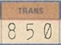TRANS: The last three digits of the assembly line and catalog part number for auto transmissions. 804 = 3515804 225 CI 806 = 3515806 318 CI 817 = 3515817 440 CI 841 = 3515841 225 CI HD 842 = 3515842 318 CI HD 843 = 3515843 340 CI 845 = 3515845 383 CI 2bbl 846 = 3515846 383 CI 4bbl 847 = 3515847 440 CI Std 848 = 3515848 440 CI HP 849 = 3515849 426 CI 850 = 3515850 440 CI 6pk 851 = 3515851 340 CI E-body 852 = 3515852 318 CI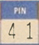PIN: The Speedo pinion gear code last two digits. 24 = 3410024 25 = 3410025 26 = 2538926 27 = 2538927 28 = 2538928 29 = 2538929 30 = 2538930 31 = 2538931 32 = 2538932 33 = 2538933 34 = 2538934 35 = 2538935 36 = 2538936 37 = 2538937 38 = 2538938 39 = 2538939 40 = 2538940 41 = 2538941 42 = 2538942 43 = 2538943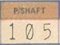P/SHAFT: The Popeller Shaft, last three digits of part number. 101 = 2996101 50.68" x 3.25" 105 = 2996109 49.50" x 3.25" 109 = 2996109 50.80" x 3.25" 113 = 2996113 53.30" x 3.25" 117 = 2996117 53.24" x 3.25" 121 = 2996121 57.42" x 3.00" 130 = 2991130 50.80" x 3.00" 186 = 2996186 53.30" x 3.00" 201 = 2996201 54.92" x 3.00" 207 = 2996207 54.92" x 3.25" 211 = 2996211 57.10" x 3.00" 216 = 2996219 57.10" x 3.00" 228 = 2996228 59.60" x 3.00" 696 = 2883696 53.18" x 3.25" 735 = 2883735 57.42" x 3.00"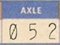AXLE: The axle code, last three digits of part number. This number is also found on the Lynch Rd Fender tag. 042 = 3.23 open, 8 3/4, 10" rear drum - front disc/LD drum 043 = 3.23 S/G, 8 3/4, 10" rear drum - front disc/LD drum 044 = 3.23 open, 8 3/4, 11" rear & front drums 045 = 3.23 S/G, 8 3/4, 11" rear & front drums 046 = 3.55 S/G, 8 3/4, 11" rear & front drums, (A36 Pkg) 047 = 3.55 S/G, 8 3/4, 10" rear drum - front disc, (A36 Pkg) 048 = 3.91 S/G, 8 3/4, 11" rear & front drums, (A31 Pkg) 049 = 3.91 S/G, 8 3/4, 10" rear drum - front disc, (A31 Pkg) 050 = 3.45 S/G, DANA 60, 11" rear & front drums, (A33 Pkg) 051 = 3.54 S/G, DANA 60, 10" rear drum - front disc, (A33 Pkg) 052 = 4.10 S/G, DANA 60, 10" rear drum - front disc, (A34 Pkg)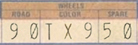WHEELS: Wheels used three boxes grouped under the heading WHEELS. The First box is for the type of wheel used on the vehicle. The Second box is the color of the wheel.NOTE: If full wheel covers are used, the the wheels would be black. If Dog Dish covers are used, in most cases the wheels would be body color. Most Spare wheels are also black unless special spare was ordered or again body color if standard hubcaps were used. The Third box is used for the type of SPARE wheel used.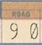WHEELS: ROAD: The last two digits of part number 50 = 2944450 15"x7" Steel Wheel 74 = 2944474 14"x5.5" Road Wheel 90 = 2944390 15"x7" Rally Wheel 78 = 3420978 14"x6" Steel Wheel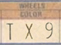WHEELS: COLOR: TX9 = Black List of Color Catalog Codes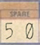WHEELS: SPARE: The last two digits of part number 50 = 2944450 15"x7" Steel Wheel 78 = 3420978 14"x6" Steel Wheel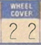WHEEL COVER: The last two digits of part number. 54 = 2944454 Standard hub cap aluminum 22 = 3461222 15" Trim ring 52 = 3461352 Rally center cap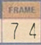FRAME: The last two digits of K-frame part number. 74 = 3583074 K-frame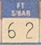FT S/BAR: The last two digits of Sway Bar part number. 62 = 2835862 .88 Dia. B-body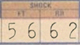SHOCK FT (Front) RR (Rear): The last two digits of factory part number. 56 = 3400556 Front B-Body HD 62 = 3402262 Rear B-Body HD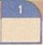1: UNKNOWN at this time for 1971.Sponsors Ads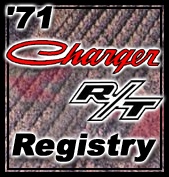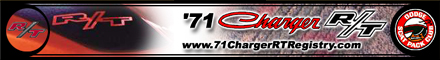71 Super Bee

"Mopar® is a registered trademark of Chrysler LLC"